## Zermelo-Fraenkel Axioms

The Zermelo-Fraenkel axioms are the basis for Zermelo-Fraenkel Set Theory. In the following,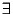stands for Exists,for is an element of,''for For All,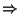for Implies,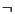for Not (Negation),for And,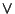for Or,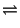for is Equivalent to,'' and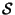denotes the unionof all the sets that are the elements of.

1. Existence of the empty set:.

2. Extensionality axiom: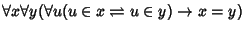.

3. Unordered pair axiom:.

4. Union (or sum-set'') axiom:.

5. Subset axiom:.

6. Replacement axiom: For any set-theoretic formula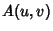,7. Regularity axiom: For any set-theoretic formula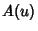,.

8. Axiom of Choice: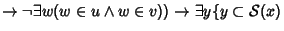9. Infinity axiom: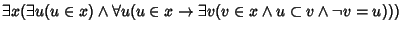.

If Axiom 6 is replaced by
6'. Axiom of subsets: for any set-theoretic formula,,
which can be deduced from Axiom 6, then the set theory is called Zermelo Set Theory instead of Zermelo-Fraenkel Set Theory.

Abian (1969) proved Consistency and independence of four of the Zermelo-Fraenkel axioms.

Abian, A. On the Independence of Set Theoretical Axioms.'' Amer. Math. Monthly 76, 787-790, 1969.
Iyanaga, S. and Kawada, Y. (Eds.). Zermelo-Fraenkel Set Theory.'' §35B in Encyclopedic Dictionary of Mathematics, Vol. 1. Cambridge, MA: MIT Press, pp. 134-135, 1980.